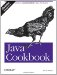# Recipe 9.2 Overriding the Equals Method

#### Problem

You want to be able to compare objects of your class.

#### Solution

Write an equals( ) method.

#### Discussion

How do you determine equality? For arithmetic or Boolean operands, the answer is simple: you test with the equals operator (==). For object references, though, Java provides both == and the equals( ) method inherited from java.lang.Object. The equals operator can be confusing, as it simply compares two object references to see if they refer to the same object. This is not the same as comparing the objects themselves.

The inherited equals( ) method is also not as useful as you might imagine. Some people seem to start their life as Java developers thinking that the default equals( ) magically does some kind of detailed, field-by-field or even binary comparison of objects. But it does not compare fields! It just does the simplest possible thing: it returns the value of an == comparison on the two objects involved! So, for any major classes you write, you probably have to write an equals method. Note that both the equals and hashCode methods are used by hashes (Hashtable, HashMap; see Recipe 7.6). So if you think somebody using your class might want to create instances and put them into a hash, or even compare your objects, you owe it to them (and to yourself!) to implement equals( ) properly.

Here are the rules for an equals( ) method:

1. It is reflexive: x.equals(x) must be true.

2. It is symmetrical: x.equals(y) must be true if and only if y.equals(x) is also true.

3. It is transitive: if x.equals(y) is true and y.equals(z) is true, then x.equals(z) must also be true.

4. It is repeatable: multiple calls on x.equals(y) return the same value (unless state values used in the comparison are changed, as by calling a set method).

5. It is cautious: x.equals(null) must return false rather than accidentally throwing a NullPointerException .

Here is a class that endeavors to implement these rules:

`public class EqualsDemo {     int int1;     SomeClass obj1;     /** Constructor */     public EqualsDemo(int i, SomeClass o) {         int1 = i;         if (o == null) {             throw new IllegalArgumentException("Object may not be null");         }         obj1 = o;     }     /** Default Constructor */     public EqualsDemo( ) {         this(0, new SomeClass( ));     }     /** Typical run-of-the-mill Equals method */     public boolean equals(Object o) {         if (o == this)                   // optimization             return true;         // Castable to this class? (false if == null)         if (!(o instanceof EqualsDemo))             return false;         EqualsDemo other = (EqualsDemo)o;    // OK, cast to this class         // compare field-by-field         if (int1 != other.int1)            // compare primitives directly             return false;         if (!obj1.equals(other.obj1))    // compare objects using their equals             return false;         return true;     } }`

And here is a JUnit test file (see Recipe 1.14) for the EqualsDemo class:

`import junit.framework.*; /** some junit test cases for EqualsDemo  * writing a full set is left as "an exercise for the reader".  * Run as: \$ java junit.textui.TestRunner EqualsDemoTest  */ public class EqualsDemoTest extends TestCase {     /** an object being tested */     EqualsDemo d1;     /** another object being tested */     EqualsDemo d2;     /** init( ) method */     public void setUp( ) {         d1 = new EqualsDemo( );         d2 = new EqualsDemo( );     }     /** constructor plumbing for junit */     public EqualsDemoTest(String name) {         super(name);     }     public void testSymmetry( ) {          assert(d1.equals(d1));     }     public void testSymmetric( ) {         assert(d1.equals(d2) && d2.equals(d1));     }     public void testCaution( ) {         assert(!d1.equals(null));     } }`

With all that testing, what could go wrong? Well, some things still need care. What if the object is a subclass of EqualsDemo? We cast it and . . . compare only our fields! You probably should test explicitly with getClass( ) if subclassing is likely. And subclasses should call super.equals( ) to test all superclass fields.

What else could go wrong? Well, what if either obj1 or other.obj1 is null ? You might have just earned a nice shiny new NullPointerException . So you also need to test for any possible null values. Good constructors can avoid these NullPointerExceptions, as I've tried to do in EqualsDemo, or else test for them explicitly.Java Cookbook, Second Edition
ISBN: 0596007019
EAN: 2147483647
Year: 2003
Pages: 409
Authors: Ian F Darwin

Similar book on Amazon